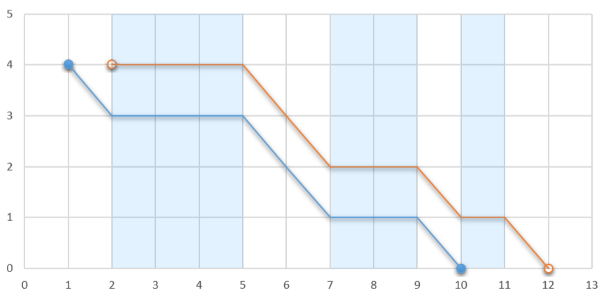# 【two-pointer】【队列】CF1041D Glider 题解

正常的two-pointer思想题

## Description

A plane is flying at a constant height of $h$ meters above the ground surface. Let’s consider that it is flying from the point $(-10^9,h)$ to the point $(10^9,h)$ parallel with $Ox$ axis.

A glider is inside the plane, ready to start his flight at any moment (for the sake of simplicity let’s consider that he may start only when the plane’s coordinates are integers). After jumping from the plane, he will fly in the same direction as the plane, parallel to $Ox$ axis, covering a unit of distance every second. Naturally, he will also descend; thus his second coordinate will decrease by one unit every second.

There are ascending air flows on certain segments, each such segment is characterized by two numbers $x_1$ and$x_2$($x_1<x_2$) representing its endpoints. No two segments share any common points. When the glider is inside one of such segments, he doesn’t descend, so his second coordinate stays the same each second. The glider still flies along $Ox$ axis, covering one unit of distance every second.If the glider jumps out at $1$, he will stop at $10$. Otherwise, if he jumps out at $2$, he will stop at $12$.

Determine the maximum distance along $Ox$ axis from the point where the glider’s flight starts to the point where his flight ends if the glider can choose any integer coordinate to jump from the plane and start his flight. After touching the ground the glider stops altogether, so he cannot glide through an ascending airflow segment if his second coordinate is $0$.

## Input

The first line contains two integers $n$ and $h(1\le n\le 2\cdot 10^5,1\le h\le 10^9)$ — the number of ascending air flow segments and the altitude at which the plane is flying, respectively.

Each of the next $n$ lines contains two integers $x_{i1}$ and $x_{i2}(1\le x_{i1}<x_{i2}\le 10^9)$ — the endpoints of the $i$-th ascending air flow segment. No two segments intersect, and they are given in ascending order.

## Output

Print one integer — the maximum distance along $Ox$ axis that the glider can fly from the point where he jumps off the plane to the point where he lands if he can start his flight at any integer coordinate.

## Examples

input
3 4
2 5
7 9
10 11
output
10

input
5 10
5 7
11 12
16 20
25 26
30 33
output
18
input
1 1000000000
1 1000000000
output
1999999999

## Note

In the first example if the glider can jump out at $(2,4)$, then the landing point is $(12,0)$, so the distance is $12-2=10$.

In the second example the glider can fly from $(16,10)$ to $(34,0)$ , and the distance is $34-16=18$.

In the third example the glider can fly from $(-100,1000000000)$ to $(1999999899,0)$, so the distance is $1999999899-(-100)=1999999999$.

## 题意：

滑翔者可以从任意一点$(x_0,h)$从飞机上跳下，$h$是给定的。给出$n$个特殊段，它们有序且互不相交。在这些段里滑翔者可以保持原来的高度，但是不能为$0$；如果不在段里，横坐标每增加$1$，纵坐标减少$1$。直到纵坐标为$0$结束。

## 题解：

这个题首先给出了有序的特殊段，且特殊段之间互不相交。很容易联想到单调队列，如果队首与队尾相差超过$h$时，就把队尾扔掉。但是在哪里保证单调性呢？经过观察，发现这里不需要单调性，只需要贪心地选最长的段就可以了。需要注意这里的段是连续的。

在没有特殊段的部分，横坐标每增加$1$，纵坐标就要减少$1$。因此第一段和最后一段之间没有特殊段的长度要小于$h$。一旦等于$h$，则最后一段的高度就是$0$，不满足题意。同时，没有特殊段的长度假设为$t$，则每次的答案为$x_{最后一段结束}-x_{第一段开始}+h-t$，其中$(h-t)$是最后一段结束时的高度，也就是最后还能自由滑下这么多距离。

而选中的段为了保证连续且最长（最长原因是尽可能悬空飞行，并且段数要尽可能多），可以用two-pointer控制前后端点之间的没有特殊段位置的长度小于$h$。这样就可以满足条件，并在每次入队时更新答案。

## Code：

#include<cstdio>
#include<cstring>
long long sum;
long long L,R;
int main()
{
int n,h,lst=0;
scanf("%d%d",&n,&h);
for(int i=1;i<=n;++i)
{
scanf("%I64d%I64d",&L[i],&R[i]);
sum[i]=sum[i-1]+L[i]-lst;
lst=R[i];
}
long long ans=0;
int t=1;
for(int i=1;i<=n;++i)
{
while(t<i&&sum[i]-sum[t]>=h)
++t;
long long tmp=sum[i]-sum[t];
ans=ans>R[i]-L[t]+(h-tmp)?ans:R[i]-L[t]+(h-tmp);
}
printf("%I64d\n",ans);
return 0;
}

### 2 说点什么0 Followers

Most reacted comment
1 Comment authorsRecent comment authors
Subscribeorz太强了,本菜鸡用lower_bound不仅码量更大还难查错…wpDiscuz